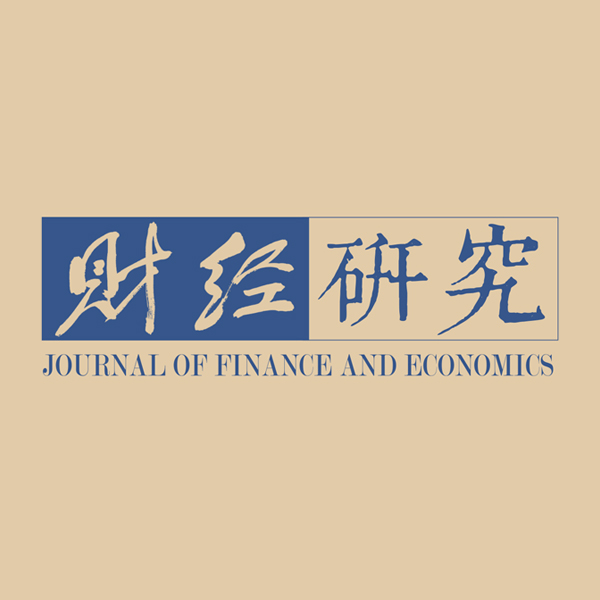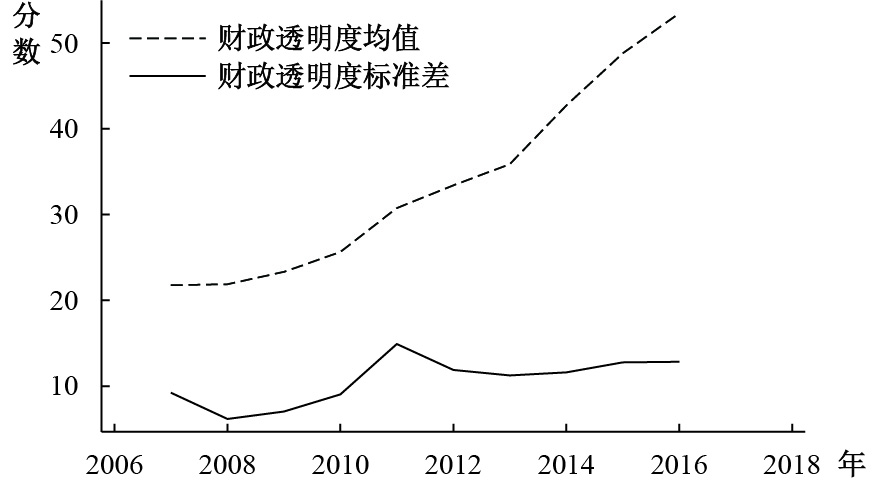﻿ 财政透明度对地方政府债务风险的影响研究《财经研究》
2019第45卷第12期

Research on the Impact of Fiscal Transparency on Local Government Debt Risks：Based on the Perspective of Intergovernmental Relations
Deng Shulian, Liu Lianyan
School of Public Economics and Administration, Shanghai University of Finance and Economics, Shanghai 200433, China
Summary: Under the general work guideline of making progress while maintaining stability, the importance and concern of local government debt risk issues are increasing. In order to alleviate the local government debt crisis, the central government has issued a number of important documents. Due to the active and continuous borrowing motives of local governments and the tendency to conceal debt behavior, the central government’s " top-down” macroeconomic control measures have little effect. Fiscal transparency is a broad " bottom-up” public monitoring tool that can effectively promote public supervision and restraint on government behavior. It has a positive and effective effect on controlling corruption, improving government credibility, and reducing debt risks. Firstly, this paper constructs a theoretical model based on game theory. The theoretical model uses the motive of local governments as the entry point to analyze how fiscal transparency will restrict local governments’ illegal debt-raising behavior. The results of the theoretical model show that: （1）With a single local government as the main player of the game, the increase in fiscal transparency will increase the expected cost of local governments’ illegal borrowing, thereby reducing the amount of illegal debt.（2）Taking two representative local governments with competitive relationship as the main players, the increase of fiscal transparency has a controlling effect on the illegal borrowing caused by inter-governmental competition. As the degree of fiscal transparency increases, the competing government will abandon the illegal way of borrowing and instead choose the legal way of borrowing.（3）With the central and local governments in the context of " Paternalism” as the main players, the improvement of fiscal transparency can strengthen the central government’s commitment to not help local governments, and encourage local governments to actively converge on illegal debt-raising in order to avoid the debt crisis. Secondly, this paper constructs an empirical model based on provincial panel data to analyze the " external performance” of the impact. The results of the study show that: （1）Improving fiscal transparency can effectively reduce local governments’ illegal borrowing impulses.（2）" Political tournaments” and " soft budget constraints” will exacerbate local governments’ debt impulses. In this context, improving fiscal transparency can effectively curb local governments’ debt impulses.（3）Improving fiscal transparency can encourage local governments to optimize their debt structure and optimize the bond issuance process. In the context of the current wide-ranging concern of local government debt problems, this paper aims to study the problem of local government debt risk control from the perspective of improving fiscal transparency. This paper provides new ideas for controlling local government debt risks, and also provides useful enlightenment for effectively promoting China’s fiscal transparency.
Key words: fiscal transparency    local government debt risks    intergovernmental relations

（一）地方政府单一主体的债务举借成本收益分析。制度特征能够显著影响个体的行为（North，1990），经济体制和政府职能的导向是形成地方政府大量举债动机的主要因素。财政分权制度形成了地方政府事权与财权不对等的格局，地方政府为了缓解在城市化进程中的财政压力及满足支出需求，不得不举债（财政部财政科学研究所，2009；缪小林和伏润民，2012；庞保庆和陈硕，2015）。同时，在我国经济发展过程中，地方政府承担了许多本该由市场解决的事务（刘尚希和赵全厚，2002），政府部门间财政过于分散且没有规范的财政监督和融资渠道（陈健夫，2013），加之地方政府官员的机会主义行为和问责机制的缺失（钟宁桦等，2018），导致地方政府滥用举债权。

$\mathop \sum \nolimits_n {X_{it}} = {X_t}$
AXit）为地方政府通过支出Xit所获得的预期收益现值，其中包括经济收益和官员获得的其他收益，通过举借债务获得额外收入能够提升当地支出能力，从而对官员晋升产生正向影响，为简化计算不区分收入来源，认为相同的财政支出产生收益的能力相同，η代表财政支出的收益能力，有
$A\left({{X_{it}}} \right) = \eta {X_{it}}$
η>1。地方政府性债务的本息之和现值为
$C1({X_{it}})$
，根据债券定价原理C1（Xit）赋予经济意义：
$C1({X_{it}}) = {X_{it}}\left[ {{{\left({1 + r} \right)}^{ - k}} + \xi \mathop \sum \nolimits_{j = 1}^k {{\left({1 + r} \right)}^{ - j}}} \right]$
，其中k为债券期限，r为折现率，ξ为债券发行利率；发行成本的现值为C2（Xit），发行成本包括经济成本、行政成本、舆论成本以及挤占发债限额的资源成本，
$C2'({X_{it}}) > 0$
，地方政府举债越多，债务可持续性风险越大，导致风险评级降低，提高发债成本。地方政府除按照中央政府规定的限额内发行政府债券合规举债外，还可能通过其他方式违规举债，在违规举债过程中，地方政府能够避免正规举债产生的发行成本C2（Xit），同时产生地方政府的议价成本σσ的大小取决于地方政府与银行或投融资平台的合作紧密程度以及官员的议价能力，此处σ小于C2（Xit），地方政府具有违规举债激励。

$P\left({TR,{X_{it}}} \right)$
，对
$P\left({TR,{X_{it}}} \right)$

$P\left({TR,{X_{it}}} \right) =$
$1 - {\left({\theta TR\times {X_{it}}} \right)^{ - Q}}$
Q>0，TR>0，Q代表财政透明度推动官员因违规行为受到处罚的作用能力，Q越大TR的影响能力越强，θ是调整系数。0≤P<1，
${P_{TR}}'(TR,{X_{it}} ) > 0$
，即透明程度越高，违规举借量越大，官员被查处的可能性越大。地方政府的违规举债行为一旦被查处，将被处以较大的惩罚F，如2017年我国对逾百涉及违法违规的官员问责。设定F为地方政府官员“成本—收益”分析中代表资金退还、罚款的可量化惩罚以及党内警告、降级甚至断送政治生涯的政绩影响的量化值。以此为基础，分析单一地方政府主体在不同财政透明度背景下的举债行为及其预期。

1. 规范发行。地方政府发行地方政府债券筹措财政债务收入，占用地方政府债券发行额度及债务限额。发债收益为

$A\left({{X_{it}}} \right)$
，发债成本为
$C1({X_{it}}) + C2({X_{it}})$
，利润为
$\varPi = A\left({{X_{it}}} \right) - C1({X_{it}}) -$
$C2({X_{it}})$

2. 违规举债。地方政府通过违规途径举借债务不占用债务限额，但地方政府存在与借债平台的议价成本及被查处风险。发债收益为AXit），发债成本为

$C1({X_{it}}) + \sigma$
，利润为
$\varPi = A\left({{X_{it}}} \right) -$
$C1({X_{it}}) - \sigma$
。在缺乏财政透明制度情况下，由于
$\sigma < C2({X_{it}})$
，地方政府有违规举债激励。

3. 财政透明背景下的违规举债。根据2014年《预算法》规定，地方政府债务信息不属于保密信息，地方政府有义务公开。在财政透明背景下，地方政府由于受到监督，其违规举债的发债成本会增加。发债收益为

$A\left({{X_{it}}} \right)$
，发债成本为
$C1({X_{it}}) +$
$\sigma + P\left({TR,{X_{it}}} \right)\times F$
，利润为
$\varPi = A\left({{X_{it}}} \right) - C1({X_{it}}) - \sigma -$
$P\left({TR,{X_{it}}} \right)\times F$

$1 > P > \hat P$
，即
$TR > {\left({\displaystyle\frac{F}{{F - C2({X_{it}}) - \sigma }}} \right)^{{{\dfrac{1}{Q}}}}} \times \left({\dfrac{1}{{\theta {X_{it}}}}} \right)$

$C2({X_{it}})$
，远小于规范举债成本
$C1({X_{it}}) +$
$C2({X_{it}})$
。然而，当财政透明度较高时，通过财政信息公开能够对官员的财政行为进行监督和追溯，官员在财政透明度较高背景下难以逃避偿债责任。

$\hat P >P >0$

 $\varPi ({X_t}) = {\rho _{1t}}{\varPi _{1t}}({X_{1t}}) + {\rho _{2t}}{\varPi _{2t}}({X_{2t}}) + {\rho _{3t}}{\varPi _{3t}}({X_{3t}}) + \cdots$ (1)

 $\varPi ({X_t}) = {\varPi _{1t}}({\rho _{1t}}{X_{1t}} + {\rho _{2t}}{X_{2t}} + {\rho _{3t}}{X_{3t}} + \cdots )$ (2)

 ${{{\varPi }}_{{{it}}}}({{{X}}_{{{it}}}}) = {{A}}\left( {{{{X}}_{{{it}}}}} \right) - {{C}}1({{{X}}_{{{it}}}}) - {{\sigma }} - {{P}}\left( {{{TR}},{{{X}}_{{{it}}}}} \right){{\times F}}$ (3)
 $A\left( {{X_{it}}} \right) = \eta {X_{it}}$ (4)
 $C1({X_{it}}) = {X_{it}}\left[ {{{\left( {1 + r} \right)}^{ - k}} + \xi \mathop \sum \nolimits_{j = 1}^k {{\left( {1 + r} \right)}^{ - j}}} \right]$ (5)
 $P\left( {TR,{X_{it}}} \right) = 1 - {\left( {\theta TR\times {X_{it}}} \right)^{ - Q}}$ (6)

 ${X_{it}} = {{{QF}}^{{\tfrac{1}{{Q + 1}}}}/\left\{{ {{{{\left( {\theta TR} \right)}}\left[ {\eta - {{\left( {1 + r} \right)}^{ - k}} - \xi \mathop \sum \nolimits_{j = 1}^k {{\left( {1 + r} \right)}^{ - j}}} \right]}}}^{{{\tfrac{1}{{Q + 1}}}}} \right\}}$ (7)

（二）地方政府间竞争：“政治锦标赛”。在政治集权、财政分权的体制背景下，我国逐渐形成了以经济指标考量官员行政能力的制度，在带动本地经济发展的同时，支出带动的经济增长方式也造成了巨额的地方政府债务和潜在的债务风险（周黎安，2007；傅勇和张晏，2007）。我们延用上述模型分析财政透明对于“政治锦标赛”导致的债务风险的控制作用。

1. 地方政府间政绩博弈的基准模型。考虑AB两省地方政府官员间的“政治锦标赛”，假设二者均具有扩大财政支出从而提高当地GDP的动力，举债是二者在短期内获得财政收入的途径之一。地方政府官员同样具有两种选择，一是在中央政府规定的限额内规范举债，二是违规举债。政绩竞争中财政支出较少的一方容易处于劣势并会因此付出一个相对额外成本D，由于政绩竞争的重要特点是一个官员的晋升会导致另一官员晋升机会降低，因此D代表官员晋升机会减少导致的损失现值。根据AB两省的不同的举债方式选择，有支付矩阵如表1所示。

 A省 B省 违规举债 规范举债 违规举债 $\underline{ A\left({{X_{it}}} \right) - C1({X_{it}}) - \sigma,}$ ${\underline{ A\left({{X_{it}}} \right) - C1({X_{it}}) - \sigma}}$ ${\underline{A\left({{X_{it}}} \right) - C1({X_{it}}) - \sigma,}}$ ${{A\left({{X_{it}}} \right) - C1({X_{it}}) - C2({X_{it}}) - D}}$ 规范举债 ${{A\left({{X_{it}}} \right) - C1({X_{it}}) - C2({X_{it}}) - D,}}$ ${\underline{A\left({{X_{it}}} \right) - C1({X_{it}}) - \sigma}}$ $A\left({{X_{it}}} \right) - C1({X_{it}}) - C2({X_{it}}),$ $A\left({{X_{it}}} \right) - C1({X_{it}}) - C2({X_{it}})$ 注：该矩阵每个单元格中第1个数组对应A省的利润，第2个数组对应B省的利润，表2−表5同。

（1）无财政透明规定。当没有财政透明规定时，由于

${{A}}\left({{X_{it}}} \right) - {{C}}1({X_{it}}) - {{\sigma }} > {{A}}\left({{X_{it}}} \right) - {{C}}1({X_{it}}) - {{C}}2({X_{it}}) >$
${{A}}\left({{X_{it}}} \right) - {{C}}1({X_{it}}) - {{C}}2({X_{it}}) - D$
，对表1使用划线法进行策略模拟可以发现，政绩竞争加剧了地方政府违规举债的倾向，由于AB两省在博弈中都存在最优决策，该支付矩阵的纳什博弈均衡为（违规举债，违规举债），即两省都会采取违规举债的行动。

（2）有财政透明规定。在财政透明制度的背景下，如前文所述，地方政府的举债行为受到监督，违规举债存在被查处和惩罚的可能。支付矩阵见表2

 A省 B省 违规举债 规范举债 违规举债 $\begin{array}{c} {\underline{A\left({ {X_{it} } } \right) - C1({X_{it} }) - \sigma - P\left({TR} \right)\times F,}}\\{\underline{ A\left({ {X_{it} } } \right) - C1({X_{it} }) - \sigma - P\left({TR} \right)\times F}} \end{array}$ $\begin{array}{c} {\underline{A\left({ {X_{it} } } \right) - C1({X_{it} }) - \sigma - P\left({TR} \right)\times F,}}\\ A\left({ {X_{it} } } \right) - C1({X_{it} }) - C2({X_{it} }) - D \end{array}$ 规范举债 $\begin{array}{c} A\left({ {X_{it} } } \right) - C1({X_{it} }) - C2({X_{it} }) - D,\\ {\underline{A\left({ {X_{it} } } \right) - C1({X_{it} }) - \sigma - P\left({TR} \right)\times F }}\end{array}$ $\begin{array}{c} A\left({{X_{it}}} \right) - C1({X_{it}}) - C2({X_{it}}),\\ A\left({{X_{it}}} \right) - C1({X_{it}}) - C2({X_{it}})\end{array}$

$A\left({{X_{it}}} \right) \!-\! C1({X_{it}}) \!-\! \sigma \!- \!P\left({TR} \right) \times F \!>\!A\left({{X_{it}}} \right) \!-\! C1({X_{it}})\! -\! C2({X_{it}})\! >$
$A\left({{X_{it}}} \right) - C1({X_{it}}) - C2({X_{it}}) - D$
，此时该矩阵存在唯一一个纳什均衡，对表2使用划线法进行策略模拟。此时，解得
$P\left({TR,{X_{it}}} \right) < \dfrac{{C2({X_{it}}) - \sigma }}{F}$
，根据C2（Xit）>σ，令
$P\left({TR,{X_{it}}} \right) =$
$\dfrac{{C2({X_{it}}) - \sigma }}{F}$
，此时TR的值为TR1。即当
$TR<TR_1$

$A\left({{X_{it}}} \right) - C1({X_{it}}) - C2({X_{it}}) > A\left({{X_{it}}} \right) - C1({X_{it}}) - \sigma - P\left({TR,{X_{it}}} \right)\times F >$
$A\left({{X_{it}}} \right) - C1({X_{it}}) - C2({X_{it}}) - D$
，解得
$\dfrac{{C2({X_{it}}) - \sigma }}{F} < P\left({TR,{X_{it}}} \right) < \dfrac{{C2({X_{it}}) + D - \sigma }}{F}$
，博弈过程见表3

 A省 B省 违规举债 规范举债 违规举债 $\begin{array}{c}{\underline{ A\left({{X_{it}}} \right) - C1({X_{it}}) - \sigma - P\left({TR,{X_{it}}} \right)\times F,}}\\ {\underline{A\left({{X_{it}}} \right) - C1({X_{it}}) - \sigma - P\left({TR,{X_{it}}} \right)\times F}} \end{array}$ $\begin{array}{c} A\left({{X_{it}}} \right) - C1({X_{it}}) - \sigma - P\left({TR,{X_{it}}} \right)\times F,\\ A\left({{X_{it}}} \right) - C1({X_{it}}) - C2({X_{it}}) - D \end{array}$ 规范举债 $\begin{array}{c} A\left({{X_{it}}} \right) - C1({X_{it}}) - C2({X_{it}}) - D,\\ A\left({{X_{it}}} \right) - C1({X_{it}}) - \sigma - P\left({TR,{X_{it}}} \right)\times F \end{array}$ $\begin{array}{c} {\underline{A\left({{X_{it}}} \right) - C1({X_{it}}) - C2({X_{it}}),}}\\ {\underline{A\left({{X_{it}}} \right) - C1({X_{it}}) - C2({X_{it}})}}\end{array}$

$A\left({{X_{it}}} \right) - C1({X_{it}}) - \sigma - P\left({TR,{X_{it}}} \right)\times F > A\left({{X_{it}}} \right) - C1({X_{it}}) - C2({X_{it}}) - D$
，因此A省同样也会选择违规举债的行为；当其预测B省选择规范举债行为时，在A省的策略集中，由于
$A\left({{X_{it}}} \right) - C1({X_{it}}) - C2({X_{it}}) >$
$A\left({{X_{it}}} \right) - C1({X_{it}}) - \sigma - P\left({TR,{X_{it}}} \right) \times F$
，所以A省同样也会选择规范举债的行为，B省的行动逻辑同理。令
$P\left({TR,{X_{it}}} \right) = \dfrac{{C2({X_{it}}) + D - \sigma }}{F}$
，此时TR的值为TR2，即
$\dfrac{{C2({X_{it}}) - \sigma }}{F} < P\left({TR,{X_{it}}} \right) < \dfrac{{C2({X_{it}}) + D - \sigma }}{F}$
$T{R_1} < TR < T{R_2}$

$T{R_1} < TR < T{R_2}$

$A\left({{X_{it}}} \right) - C1({X_{it}}) - C2({X_{it}}) >A\left({{X_{it}}} \right) -$
$C1({X_{it}}) - \sigma - P\left({TR} \right) \times F$
，两个纳什均衡中博弈双方以及社会收益最高的选择是（规范举债，规范举债）。我们可以认为（规范举债，规范举债）是这个博弈中的帕累托上策均衡。由此，博弈双方未必一定会达成共同规范举债的共识。例如，在政绩竞争中落后导致的额外成本D较大时，虽然地方政府之间可以协商共同采取规范举债的措施，但是如果约定的一方选择背叛约定，则另一方的损失将会非常巨大，为了避免这样的情况出现，双方都会放弃规范举债的选项，转而选择没有风险的违规举债策略，（违规举债，违规举债）是该博弈的风险上策均衡，此时会造成更高的负外部性和社会成本。在这种情况下，为在政绩竞争中落败的一方提供举报违规举债的通道也许是一种解决方式。

$A\left({{X_{it}}} \right) - C1({X_{it}}) - C2({X_{it}}) > A\left({{X_{it}}} \right) - C1({X_{it}}) - C2({X_{it}}) - D >$
$A\left({{X_{it}}} \right) - C1({X_{it}}) - \sigma - P\left({TR,{X_{it}}} \right)\times F$
，解得
$P\left({TR,{X_{it}}} \right) < \dfrac{{C2({X_{it}}) + D - \sigma }}{F}$
，此时
$TR > T{R_2}$
，博弈过程见表4

 A省 B省 违规举债 规范举债 违规举债 $\begin{array}{c} A\left({ {X_{it} } } \right) - C1({X_{it} }) - \sigma - P\left({TR,{X_{it} } } \right)\times F,\\ A\left({ {X_{it} } } \right) - C1({X_{it} }) - \sigma - P\left({TR,{X_{it} } } \right)\times F \end{array}$ $\begin{array}{c} A\left({ {X_{it} } } \right) - C1({X_{it} }) - C2({X_{it} }) - D,\\{\underline{ A\left({ {X_{it} } } \right) - C1({X_{it} }) - \sigma - P\left({TR,{X_{it} } } \right)\times F}} \end{array}$ 规范举债 $\begin{array}{c} {\underline{A\left({ {X_{it} } } \right) - C1({X_{it} }) - \sigma - P\left({TR,{X_{it} } } \right)\times F,}}\\ A\left({ {X_{it} } } \right) - C1({X_{it} }) - C2({X_{it} }) - D \end{array}$ $\begin{array}{c} {\underline{A\left({{X_{it}}} \right) - C1({X_{it}}) - C2({X_{it}}),}}\\ {\underline{A\left({{X_{it}}} \right) - C1({X_{it}}) - C2({X_{it}})}}\end{array}$

（三）中央政府与地方政府间博弈：“预算软约束”。“预算软约束”根源于国家或政府的责任归属问题（林毅夫和谭国富，2000），是造成地方政府债务违约风险的关键（王永钦等，2016）。具体来说就是在央地的“委托–代理”关系中，一方面，中央政府考虑到地方政府承担了大量的支出责任，会在一定程度上“默许”地方政府增加“隐性债务”；另一方面，“父爱主义”特征使得中央政府认为自己有责任对地方政府进行救助（Kornai，1980）。在分税制度下，中央政府的“预算软约束”会降低其对地方政府“不救助”信号的可信度，而中央转移支付的“公共池”属性也会使得地方政府产生增加举债额的意愿（郭玉清等，2016），使得偿债责任和债务风险从地方政府向中央政府，甚至向金融系统转嫁。

$G\left({{X_{it}}} \right)$
，为了满足支出需求，地方政府的违规融资冲动仍然存在，当违规融资达到一定数额Xkt时将会带来财政不可持续风险，债务违约累积的结果便是债务风险的爆发，若中央政府不予施救，不仅无法获得治理收益
$G\left({{X_{kt}}} \right)$
，地方和中央还要分别为防范当期债务风险的爆发付出极大的成本RLtRCt，有
$R_t=$
$RL_t+RC_t$
，主要用于调整财政收支，激活断裂资金链、恢复经济景气等。如果中央政府选择采取救助手段，其救助成本为
$GC\left({{X_t}} \right)$
，由于防范即将爆发的风险的总成本
$R_t$

$\left| {GC\left({{X_t}} \right)} \right| < \left| {{R_t}-G\left({{X_{kt}}} \right) } \right|$
。地方政府与中央政府间博弈的支付矩阵见表5

 地方 中央 违规举债 规范举债 事后不救助 $\begin{array}{c} - R{C_t},\\ A\left({{X_{kt}}} \right) - C1\left({{X_{kt}}} \right) - \sigma - R{L_t}\end{array}$ $\begin{array}{c} G\left({ {X_{kt} } } \right),\\ A\left({ {X_{kt} } } \right) - C1({X_{kt} }) - C2\left({ {X_{kt} } } \right)\end{array}$ 事后救助 $\begin{array}{c} G\left({{X_{kt}}} \right) - CG\left({{X_t}} \right),\\ A\left({{X_{kt}}} \right) - C1\left({{X_{kt}}} \right) - \sigma \end{array}$ $\begin{array}{c} G\left({ {X_{kt} } } \right),\\ A\left({ {X_{kt} } } \right) - C1({X_{kt} }) - C2({X_{kt} })\end{array}$

$- {R_t} < G\left({{X_{kt}}} \right) - CG\left({{X_t}} \right)$
，因此中央政府不救助的承诺缺乏可信性，地方政府判断中央政府一定会对其救助，因此对违规举债无所顾忌。

（一）实证对象的选择。本文选取我国的融资平台债务和城投债券数据作为实证对象，主要由于：（1）地方政府债务信息的透明度较低，长期数据的获得较为困难，本文关注的平台债和城投债数据较为直接。（2）平台债和城投债具有地方政府性债务属性，是我国地方政府性债务的主要组成部分。由于早期地方政府债券发行受限，地方政府主要借助地方融资平台通过银行贷款和发行“城投债”的方式获得资金（魏加宁，2004），有学者认为，城投债发挥了外国市政债券的作用，实际上就是中国的市政债券（周沅帆，2010）。（3）城投债对于财政透明度具有较高的敏感度。持有市政债券的居民出于保护持有债券价值的目的，有权利并倾向于要求地方政府披露更多信息（马骏，2014），因此城投债券相关指标对于财政透明度的敏感性较高。

（二）数据来源与变量选择。

1. 因变量选取。本文因变量数据来源于Wind数据库，以2007—2017年期间Wind口径的融资平台债务以及融资平台发行城投债券的年度数据作为研究样本。融资平台债务包括平台公司的流动负债（短期借款，一年内到期的非流动负债）和非流动负债（长期借款和应付债券）；城投债券数据包含了发行规模、发行利率、发行主体地域、发行主体行业和发行主体信用评级等信息。在数据处理上，我们将各省的省级、地级市及以下地方融资平台均识别为该省融资平台，其平台债务加总成为该省融资平台债务总额数据，由于数据可得性问题，没有考虑西藏自治区的情况。我们选取了融资平台债务总额、城投债券发行额占融资平台债务的比重、各地债券主体评级的平均值、各地债券利率的平均值这几个变量作为从不同角度衡量城投债风险的被解释变量。

2. 自变量选取。用上海财经大学公共政策研究中心《中国财政透明度报告》中对我国省级财政透明度的评分度量财政透明度（trans）。报告发布时间为2009年至2018年，报告的发布滞后两年，数据时间为2007年至2016年。我国的省级财政透明度得分呈现同一地区分数波动较大，均值稳步上升，标准差波动较大的趋势，详见图1图 1 省级财政透明度情况

3. 控制变量选取。从社会经济状况、社会投资状况和财政收支状况三个角度选取控制变量，数据主要来源于国家统计局、各省统计年鉴和Wind数据库。根据被解释变量类别对控制变量进行调整，被解释变量从总量描述变更为比例描述时，控制变量也随之调整为相应的比例描述变量。其中社会经济状况变量包括：城市人口数（城市化率）、地区生产总值（地区人均生产总值）、第三产业增加值（第三产业增加值占GDP的比重）；社会投资状况变量包括：外商直接投资（人均外商直接投资）、全社会固定资产投资（全社会固定资产投资占GDP的比重）；财政收支状况变量包括：社保余额（社保余额占GDP比重）和财政赤字（财政赤字率）。

（三）模型设定。为了验证上文提出的假设，我们对财政透明度与地方政府债务相关指标进行面板模型回归。为了克服估计有偏，控制了个体和时间固定效应。由于地方政府每年的预决算一般集中在4月及11月公开，当年的财政信息公开情况可能难以对当年的决策形成影响，因此将自变量与控制变量滞后一期，设定回归模型（8）：

 ${y_{it}} = {\beta _0} + {\beta _1}tran{s_{i\left( {t - 1} \right)}} + {X_{i\left( {t - 1} \right)}}{\beta _2} + {\mu _i} + {\varphi _t} + {\varepsilon _{it}}$ (8)

$tran{s_{i\left({t - 1} \right)}}$

${X_{i\left({t - 1} \right)}}$

（四）实证结果。

1. 基准回归。本文使用面板固定效应模型（FE）研究财政透明度对地方政府融资平台债务总额的直接影响，结果在表6的回归（1）中报告。为了检验财政透明度对处在“政绩竞争”背景中的地方政府的作用效果，根据省际的地理条件构造相邻矩阵，以该省相邻省份GDP增长率的平均值来刻画其面临的“竞争压力”，将每年所有地区“竞争压力”的中位数作为临界值，把变量“地区间竞争”设置为二元函数，将“竞争压力”大于临界值地区的“地区间竞争”赋值为1，其余为0。为了检验财政透明度对于处在“预算软约束”背景中的地方政府的作用效果，以中央对省的转移支付总量代表中央对地方政府的救助倾向，地方收到的转移支付越多，预算约束越为软化，将每年所有地区转移支付额的中位数作为临界值，把变量“转移支付”设置为二元函数，将转移支付额大于临界值地区的“转移支付”赋值为1，其余为0。我们在表6的回归（2）中加入以上两个指标，为了与解释变量一致，加入的指标均滞后一期。

 变量 （1）FE （2）FE （3）FE （4）FE （5）FE debt debt issueratio rank rate 财政透明度 −45.53***（14.06） −40.37***（13.95） 0.0480**（13.99） 0.0282**（13.99） −0.00809**（13.95） 地区间竞争 611.8**（300.9） 转移支付 1 120*（600.3） 控制变量 Y Y Y Y Y 省级/年度 Y Y Y Y Y Observations 300 300 300 300 279 R-squared 0.762 0.771 0.441 0.296 0.691 注：*、**、***分别代表在10%、5%、1%的显著水平，括号中的数值为标准差，表内解释变量及所有控制变量均滞后一期，下表统同。

2. 稳健性检验。即使考虑了所有控制变量，被解释变量与解释变量间的内生性影响仍有可能导致估计的偏误。我们使用Heckman两阶段估计减轻样本选择偏误导致的内生性问题，在Heckman两阶段估计的第一阶段，我们构建了影响融资平台债务总量的两值模型，两值因变量使用指标“平台债务程度”表示，将融资平台债务总量位于平均线以上样本的指标赋值为1，其余赋值为0，第一阶段的控制变量加入了代表当地教育水平的受高中及以上教育人口占比（midsch），第二阶段主回归的自变量和控制变量与基准回归相同，回归结果在表7的回归（1）和（2）中报告。Heckman检验中，财政透明度对地方政府城投债券发行量占融资平台债务比重的逆米尔斯率（Mill’s ratio）显著，表明样本存在因选择偏误导致的内生性问题，其根源可能是对城投债券发行、偿还和风险控制等较为科学、高效的政府更愿意接受公众的监督。Heckman检验的第二阶段将计算得到的逆米尔斯率作为控制变量，用于控制可能的样本选择偏误，第二阶段后回归（1）和（2）的检验结果与基准回归一致，分别在1%和5%的水平上显著，这说明基准回归结论具有稳定性。

 变量 （1）debt （2）issueratio main 财政透明度 −112.8***（24.22） 0.0454**（0.0103） 教育水平 −16.86***（2.465） −24.24***（3.249） 控制变量 Y Y mills lambda −676.2（1 083） 1.556**（0.781） Observations 270 270

 变量 （1） debt（IV） （2） issueratio（IV） （3） debt（FE） （4） issueratio（FE） 财政透明度 −243.7**（107.5） 0.378**（0.189） −18.47*（9.824） 0.0845*（0.0506） 一阶段F值 21.77 21.5 控制变量 Y Y Y Y 省级/年度 Y Y Y Y Observations 270 270 300 298 R-squared 0.519 −0.137 0.531 0.221

3. 异质性分析。地方政府举债的重要目的是缓解财政支出压力，我们根据地方政府财政赤字的程度构造虚拟变量“财政赤字水平”，并将年平均财政赤字规模大于均值的地区赋值为1，其余为0，通过构造交乘项的方式，分析财政透明度作用的地区异质性，结果在表9的回归（1）和（2）中报告。从回归结果可以看出，赤字水平较高的地区被解释变量的系数符号、显著性与基准回归基本一致，赤字水平较低的地区，财政透明度对地方政府债务总额和结构没有显著规范作用。结论与上文理论模型结论一致，理论模型中，概率函数

$P\left({TR,{X_{it}}} \right)$

$P\left({TR,{X_{it}}} \right)$

 变量 （1）debt （2）issueratio （3）debt_current （4）debt_non 财政透明度 −8.075（19.09） −0.00875（0.0313） −13.79***（3.513） −31.74***（10.89） 财政赤字水平×财政透明度 −59.86***（20.35） 0.0750**（0.0326） 控制变量 Y Y Y Y 省级/年度 Y Y Y Y Observations 300 300 300 300 R-squared 0.763 0.434 0.695 0.770

2018年底的中央经济工作会议明确了打好防范化解重大风险攻坚战，稳妥处理地方政府债务风险的目标。财政部2018年12月18日印发的《地方政府债务信息公开办法（试行）》对切实提高地方政府债务信息透明度，防范地方政府债务风险提出了具体要求。在各界广泛关注地方政府债务问题的背景下，本文从提升财政透明度的角度研究了我国地方政府债务风险控制问题，首先构建理论模型进行“内因”的研究，较为全面地研究了财政透明度对不同政府间关系引致的地方政府债务风险的影响。理论模型表明：（1）财政透明引入了对地方政府的长效监督机制，提高了地方政府违规借债的预期成本，从而使得地方政府违规举债动力下降；（2）较高的财政透明度能够在一定程度上抑制政府间无序的“支出竞争”，引导地方政府间达成共同遵守地方债法规的策略；（3）财政透明度的提高能够硬化中央对地方的“预算软约束”，使得地方由于预期到中央的救助机会渺茫而主动规范债务行为。其次，我们构建了一个基于省级面板数据的实证模型，分析该影响的“外在表现”。实证检验结果表明，随着财政透明度的提升，地方政府会迫于压力而从多个角度规范地方债的风险管理，削减违规举债规模，优化债务结构以及强化发债过程管控，能使地方财政的运行步入更健康的轨道。

① 财政部2013年发布的《地方政府自行发债试点办法》规定了地方政府债券的定价机制，即采用单一利率发债机制确定债券发行利率。2015年印发的《地方政府一般债券发行管理暂行办法》规定，一般债券的定价机制和发行利率采用承销和招标等方式确定。本文将地方政府发行的债券都视为单一利率，每年付息，到期还本。

② 限于篇幅，本文描述性统计表格未列出，详情可参见《财经研究》“工作论文”（WP2019-0047）。

③ “划线法”通过对每个策略的最佳收益对策添加下划线来进行策略模拟，博弈结果的纳什均衡存在于每个组下都有短线的策略组合。

  邓淑莲. 财政公开透明: 制度障碍及破阻之策——基于对我国省级财政透明度的7年调查和评估[J]. 探索, 2016(3): 62–68. DOI:10.3969/j.issn.1005-8095.2016.03.014  傅勇, 张晏. 中国式分权与财政支出结构偏向: 为增长而竞争的代价[J]. 管理世界, 2007(3): 4–12，22.  郭玉清, 何杨, 李龙. 救助预期、公共池激励与地方政府举债融资的大国治理[J]. 经济研究, 2016(3): 81–95. DOI:10.3969/j.issn.1005-2674.2016.03.011  黄寿峰, 郑国梁. 财政透明度对腐败的影响研究——来自中国的证据[J]. 财贸经济, 2015(3): 30–42.  蒋洪. 现代财政/预算制度在中国: 进展与挑战[J]. 探索, 2016(3): 57. DOI:10.3969/j.issn.1672-0423.2016.03.041  刘尚希, 赵全厚. 政府债务: 风险状况的初步分析[J]. 管理世界, 2002(5): 22–32.  马骏. 以市政债制度硬化地方政府预算约束[J]. 新金融, 2014(7): 14–16.  上海财经大学公共政策研究中心. 2017中国财政透明度报告[M]. 上海: 上海财经大学出版社, 2017: 11.  孙琳, 陈舒敏. 债务风险、财政透明度和记账基础选择——基于国际经验的数据分析[J]. 管理世界, 2015(10): 132–143.  王永钦, 陈映辉, 杜巨澜. 软预算约束与中国地方政府债务违约风险: 来自金融市场的证据[J]. 经济研究, 2016(11): 96–109. DOI:10.3969/j.issn.2095-3151.2016.11.021  谢作诗, 李善杰. 软预算约束的原因与性质: 一个改进的一般化模型[J]. 经济学(季刊), 2015(3): 1193–1210.  张维迎. 博弈与社会[M]. 北京: 北京大学出版社, 2013.  钟宁桦, 汪峰, 张牧扬. 城投债扩张: 晋升压力与终身问责缺失[R]. 北京: 组织经济学Seminar, 2018. http://www.pinggu.org/index.php?m=content&c=index&a=show&id=3928807&page=xy  周黎安. 晋升博弈中政府官员的激励与合作——兼论我国地方保护主义和重复建设问题长期存在的原因[J]. 经济研究, 2004(6): 33–40. DOI:10.3969/j.issn.1004-9746.2004.06.012  周沅帆. 城投债−中国式市政债券[M]. 北京: 中信出版社, 2010.  Alesina A, Perotti R. Fiscal discipline and the budget process[J]. The American Economic Review, 1996, 86(2): 401–407.  Alt J E, Lassen D D. Fiscal transparency, political parties, and debt in OECD countries[J]. European Economic Review, 2006, 50(6): 1403–1439. DOI:10.1016/j.euroecorev.2005.04.001  Benito B, Bastida F. Budget transparency, fiscal performance, and political turnout: An international approach[J]. Public Administration Review, 2009, 69(3): 403–417. DOI:10.1111/j.1540-6210.2009.01988.x  Hameed F. Fiscal transparency and economic outcomes[R]. IMF Working Papers No.05/225, 2005.  Kornai J. Economics of shortage[M]. Amsterdam: North-Holland Pub Co, 1980.  Lu Y Q, Sun T. Local government financing platforms in China: A fortune or misfortune? [R]. IMF Working Papers No.13/243, 2013. https://papers.ssrn.com/sol3/papers.cfm?abstract_id=2375564  Oet M V, Bianco T, Gramlich D, et al. SAFE: An early warning system for systemic banking risk[J]. Journal of Banking & Finance, 2013, 37(11): 4510–4533.